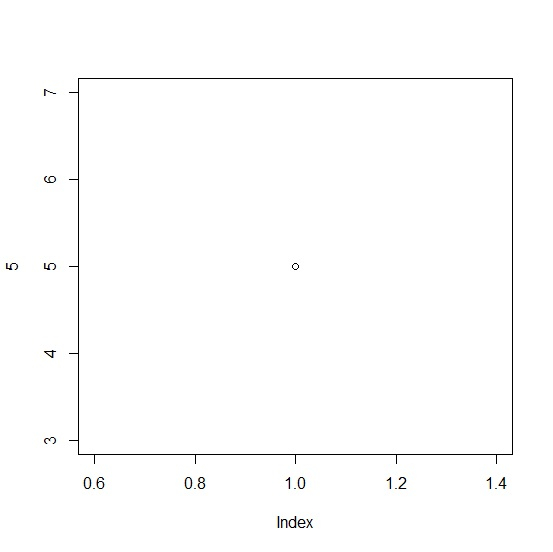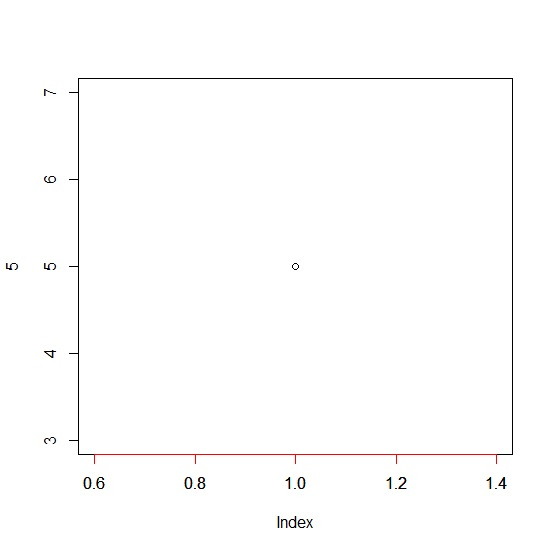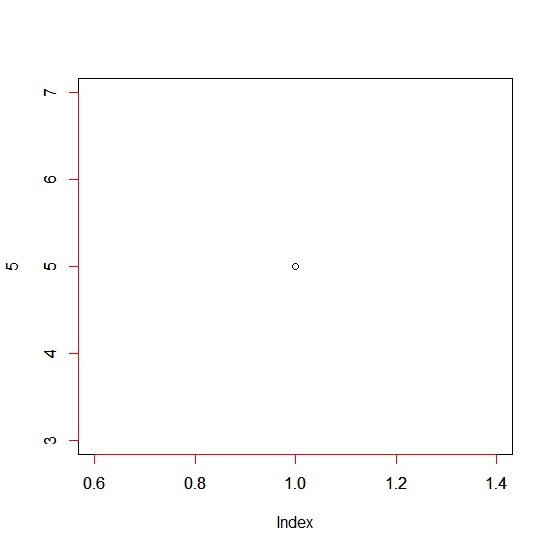# How to change the axis color in base R plot?

To change the axis color in base R plot, we can use axis function after creating the plot.

For example if we want to change the color of X-axis to blue then we can use the command given below −

axis(1,col="blue")

If we want to change the color of Y-axis to red then, we can use the command given below −

axis(2,col="blue")


Check out the below example to understand how it works.

## Example

To change the axis color in base R plot, use the code given below −

plot(5)

## Output

If you execute the above given code, it generates the following output −To change the axis color in base R plot, add the following code to the above snippet −

plot(5)
axis(1,col="red")

## Output

If you execute all the above given codes as a single program, it generates the following output −To change the axis color in base R plot, add the following code to the above snippet −

plot(5)
axis(2,col="red")

## Output

If you execute all the above given codes as a single program, it generates the following output −